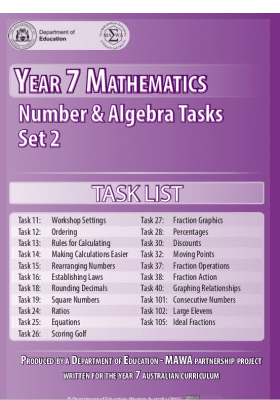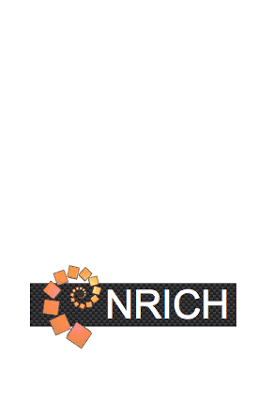# Resources for Year 7 students

## Resources for Year 7 students

Resources are available across all year levels and learning areas, to give children and young people the best opportunity to continue to learn at home.

## Aset Penerbit

•### Number and Algebra - Commutative law and subtraction and division

•### Number and Algebra - Commutative law for addition

•### Number and Algebra - Commutative law for multiplication

•### Number and Algebra - Dividing fractions

•### Number and Algebra - Equivalent fractions and the use of the number line

•### Number and Algebra - Laws of arithmetic

•### Number and Algebra - Multiplication and division of decimals

•### Number and Algebra - Multiplying and dividing mixed numerals

•### Number and Algebra - Multiplying fractions

•### Number and Algebra - Notation

•### Number and Algebra - Pronumerals

•### Number and Algebra - Square roots and square numbers

•### Number and Algebra Task List - Set 1

•### Number and Algebra Task List - Set 2

•### Number and Algebra Task List - Set 3

•### Number and Algebra- Expressing one quantity as a fraction of a second

Read the information about expressing one quantity as a fraction of a second and answer the questions.

•### Number and Algebra- Rounding decimals

•### Orange drink

A problem solving task exploring proportional reasoning

•### Percentages

This resource should take you approximately two weeks to complete. It comprises seven learning sections, a summary section and a review task section.

•### Printable templates for Mathematics

Access useful printable items for maths at home such as grid paper, dot paper and number cards

— 20 Item per Halaman
Menampilkan 41 - 60 hasil 88

Clear